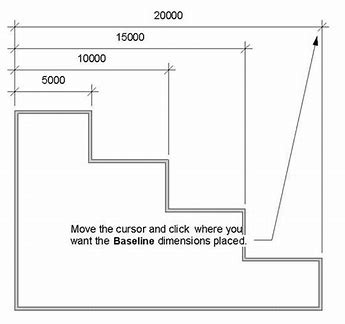# Placing DimensionLinear object in the middle of a rectangle

I would like to display a `Sketchup::DimensionLinear` object right in the middle of a rectangle. For example, the following code:

``````ents = Sketchup.active_model.entities
ents.add_face([0, 0], [100, 0], [100, 200], [0, 200])
ents.add_dimension_linear([50, 0, 0], [50, 200, 0], [1, 0, 0])
ents.add_edges([50, 0, 0], [50, 200, 0])
``````

creates a rectangle face with a vertical edge in the middle, but the dimension is offset a little bit to the right.

Is it possible to display the dimension on the vertical axis of this rectangle without having to adjust the endpoints? Changing `[50, 0, 0]` and `[50, 200, 0]` to `[49, 0, 0]` and `[49, 200, 0]` does the trick, but that feels more like a hack than a proper solution.

Because the length of the offset vector (which you supplied as the 3rd argument to `add_dimension_linear`,) is `1` inch and points in the `x` direction.

The docs describe the offset vector as:

• offset_vector (Geom::Vector3d) —
the parallel offset vector from the reference line to the dimension line measured from the ‘start’ reference point.

Well the obvious question for this case would be:

• Does the factory method accept a zero length offset vector argument ?

Trying …

``````ents.add_dimension_linear([50, 0, 0], [50, 200, 0], [0, 0, 0])
``````

… produces …

``````#<ArgumentError: Invalid elements in the array.>
``````

… so the answer is no.

The closest you can get is:

``````ents.add_dimension_linear([50, 0, 0], [50, 200, 0], [0.001, 0, 0])
``````
1 Like

Oh, wow. I don’t know why I was under the impression that the 3rd argument must be a vector of magnitude 1… Thanks again, Dan!

1 Like

If you are adding a series of dimensions from the same baseline, then each offset would be larger than the previous (according to whatever drafting standard is applied.)